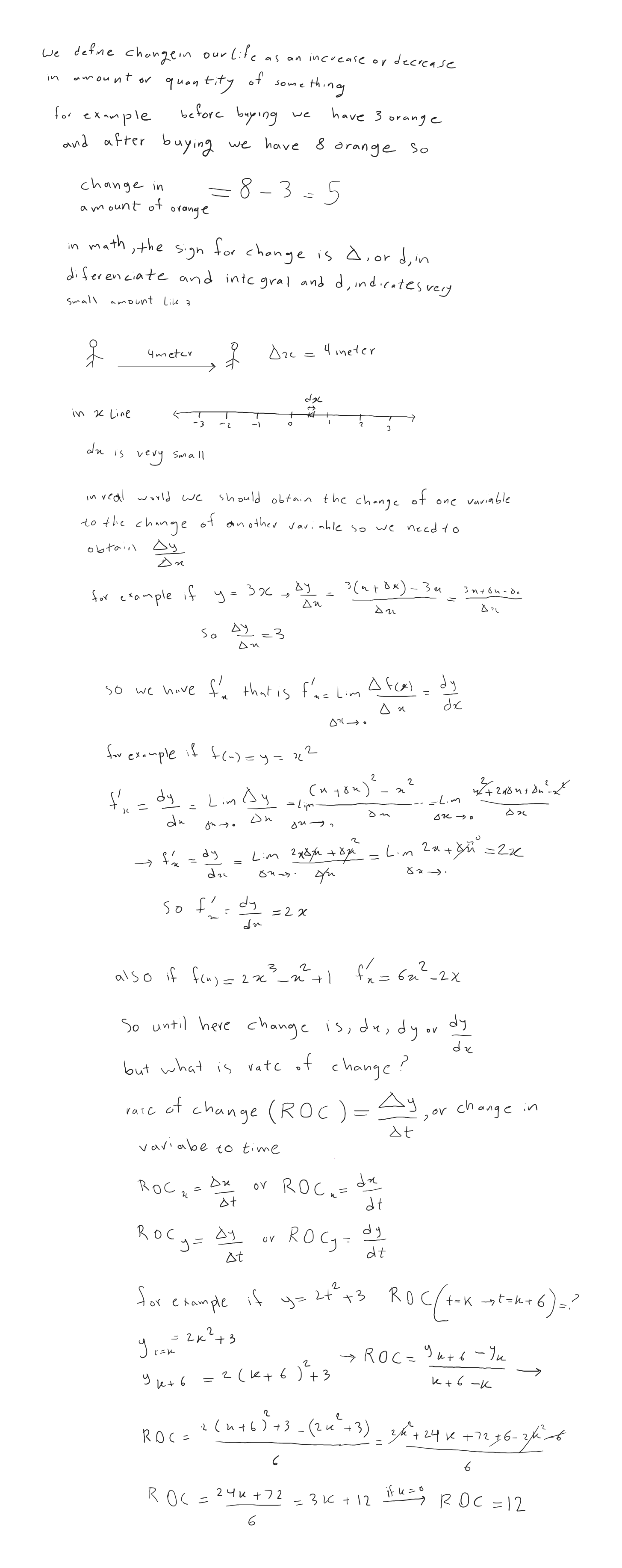SherpaMaths

>

GCSE

Rates of Change

Question

# How do you find the rate of change?

1 year ago

·

128 Replies

·

4482 views

V

Vickie Shanahan

A
Archita Sinha

The rate of change on a line graph is called the gradient and is calculated by the change in y axis/ change in x axis. What this means is that you find 2 points on the line and find the difference between the y coordinates and divide that by the difference in x axis.

I
Iliirjan

The rate of change in measured the change in Y over the change in X

D
Darcy Peake

The rate of change refers to how much something for example distance is changing in a specific unit of time. In order to work out the rate of change, you must divide the thing that is changing with how much it has changed in such a unit of time.

T
Timothy M Lewis

Assuming rate of charge is the rate of flow of charge, this is known as current (I). This can be found by dividing charge (Q) by time (t) - I = Q/t

P
Paul Hunt

This is easier to work out from a graph, the rate of change is the gradient of the line - the change in y divided by the change in x. However, you might not have time in exam conditions to draw a graph when it's not asked of you, so I would suggest picking two points of the data - ignoring outliers if there are any. Calculate the difference between them by taking them away from each other, then divide that by the difference in time between them. This will give you the rate of change.

Vashisht K Verified Sherpa Tutor ✓

4 years teaching GCSE. Engineering Student at King's College London

2 reviews

In a graph of a value vs time, it would be the gradient. Otherwise simply divide the difference in any particular value by the time taken.

I'm available for 1:1 private online tuition!

DR M Verified Sherpa Tutor ✓

An experienced tutor for science subjects and competitive exams

If you take the change in Y values and divide that with the change in X-values the result will be a rate of change,

I'm available for 1:1 private online tuition!

S
Sonya Pervez

The rate of change (ROC) is how much the value of something changes over a period of time and it is given in percentage terms.

To find this you first calculate the change in "Y" values (or the change in an outcome variable) divided by the change in "X" values (or the change in input variables).

So if my tutoring time increases from 3 hours in week 1 to 16 hours in week 2 and my income increases from £45 per week to £240 per week, Y values are my income and X values are the work hours I put in. r

To calculate the ROC I would do the following.

(240 - 45) / (16 - 3) = 195 / 13 = 15% rate of change in my earnings between week 1 and week 2.

Emanuel Verified Sherpa Tutor ✓

Experienced and enthusiastic Maths Tutor and Maths teacher of GCSE and

You would have to differentiate

I'm available for 1:1 private online tuition!

S
Stavros Savva

Divide the change of one variable with the change of the other variable

A
Angie Muyeke

The rate of change can be found on a straight-line graph by selecting two coordinates and dividing the change in the y-values by the change in the x-values. This can be shown in the formula : m = y2-y1/ x2-x1 with m representing the gradient of the straight-line. Additionally, x and y can be two different variables and the formula is used to calculate average speed or average velocity.The rate of change of a straight line is equivalent to the gradient of a straight line graph. However, we use differentiation with non-linear graphs to find the rate of change at a particular point. This is another topic in itself.

M
Milton Tom

Frays of change is how much x. Ganges w time or another variable . So this can be done by working out the gradient of a graph or differentiating If it is an expression

S
Soufyan Soogun

Find the change in y, and divide it by the change in x!

For example...

On a graph, we may find two points (5,4) and (6,8).

We can calculate rate of change by taking the x values (5 and 6), and finding the difference between them (6-5=1). We do the same for the y values (4 and 8), with the difference being 4.

Therefore, the rate of change = 4 / 1 = 4!

F
Farooq

The rate of change (ROC) is the speed at which a variable changes over a specific period of timeElizabeth Verified Sherpa Tutor ✓

4 reviews

divide the change in y-values by the change in x-values

I'm available for 1:1 private online tuition!